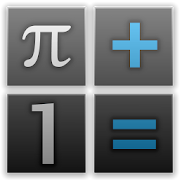# Prime CalcEveryone
684
PrimeCalc - free scientific symbolic calculator

- 70 functions, 20 mathematical constants and up to six variables
- New functions and constants can be defined by the user
- Supports complex numbers
- Matrices, vectors, sets and calculations on them
- Solves the mathematical equations
- Calculate the integrals and derivatives
- Step-by-step calculations
- And many more

Supported functions, operators, and mathematical constants:

Arithmetic:
Operators +, -, ×, ÷, %
x^n - nth power of x
√(x) - Square root of x
√(n, x) - nth root of x
ln(x) - Natural logarithm of x
log(x) - Logarithm of x to base 10
log(n, x) - Logarithm of x to base n
∑(f(x), imin, imax) - Summation of f(x) from imin to imax
∏(f(x), imin, imax) - Product of f(x) from imin to imax

Mathematical analysis:
∫(f(x)) - Indefinite integral of f(x)
∫(f(x), xmin, xmax) - Definite integral of f(x) from xmin to xmax
∂(f(x)) - Derivative of f(x)
lim(f(x), c) - Limit of f(x) when x approaches c

Equation solving:
Representation of polynomial - x^2+3x-2=0
= - Polynomial equation operator

Numerical Functions:
m mod n - Remainder of m ÷ n
gcd(m, n) - Greatest common divisor of m and n
lcm(m, n) - Least common multiple of m and n
abs(n) - Absolute value of n
round(n) - Integer closest to n
frac(n) - Fractional part of n
floor(n) - Floor value of n
ceil(n) - Ceiling value of n

Complex numbers:
Representation - 5+2i
re(c) - Real part of complex number c
im(c) - Imaginary part of complex number c

Statistics:
median([a]) - Median of [a]
gmean([a]) - Geometric mean of [a]
amean([a]) - Arithmetic mean of [a]
randi(n) - Random integer from 0 to n
randr - Random real from 0 to 1

Number theory:
harmonicN(n) - nth harmonic number

Combinatorics:
n! - Factorial of n
binomial(n, k) - Binomial coefficient
multinomial(n1, n2, ...) - Multinomial coefficient
catalanN(n) - nth Catalan number
fibonacci(n) - nth Fibonacci number

Trigonometric functions:
sin(x), cos(x), tan(x)
sec(x), csc(x), cot(x)
asin(x), acos(x), atan(x), acot(x)

Hyperbolic functions:
sinh(x), cosh(x), tanh(x)
arsinh(x), arcosh(x), artanh(x)

Matrixes:
Representation - [[1,2],[3,4]]
[m1]⋅[m2] - Product of [m1] and [m2]
tran([m]) - Transpose [m]
ctran([m]) - Conjugate and transpose [m]
inverse([m]) - Invert [m]
det([m]) - Determinant of [m]
tr([m]) - Trace of [m]
mpow([m], n) - nth matrix power of [m]

Number sets:
Representation - [1,2]
union([a1], [a2]) - Union of two sets
intersec([a1], [a2]) - Intersection of two sets
max([a]) - Largest element of [a]
min([a]) - Smallest element of [a]

Constants:
π - Number Pi
e - Euler's number
i - Imaginary unit
∞ - Infinity
γ - Euler–Mascheroni constant
G - Catalan's constant
A - Glaisher–Kinkelin constant
φ - Golden ratio
κ - Khinchin's constant
C₂ - Twin prime constant
ζ₃ - Apéry's constant
B₄ - Brun's constant for prime quadruplets
B₂ - Brun's constant for twin primes
EB - Erdős–Borwein constant
δ - Feigenbaum first constant
α - Feigenbaum second constants
BL - Legendre's constant
M₁ - Meissel–Mertens constant
Collapse

## Reviews

Review policy and info
4.7
684 total
5
4
3
2
1

## What's New

Memory support (M+ and M buttons)
History support ("History" from main menu)
Minor fixes
Collapse

Updated
September 20, 2013
Size
1.7M
Installs
10,000+
Current Version
0.6
Requires Android
2.1 and up
Content Rating
Everyone
Permissions
Offered By
Aeiou